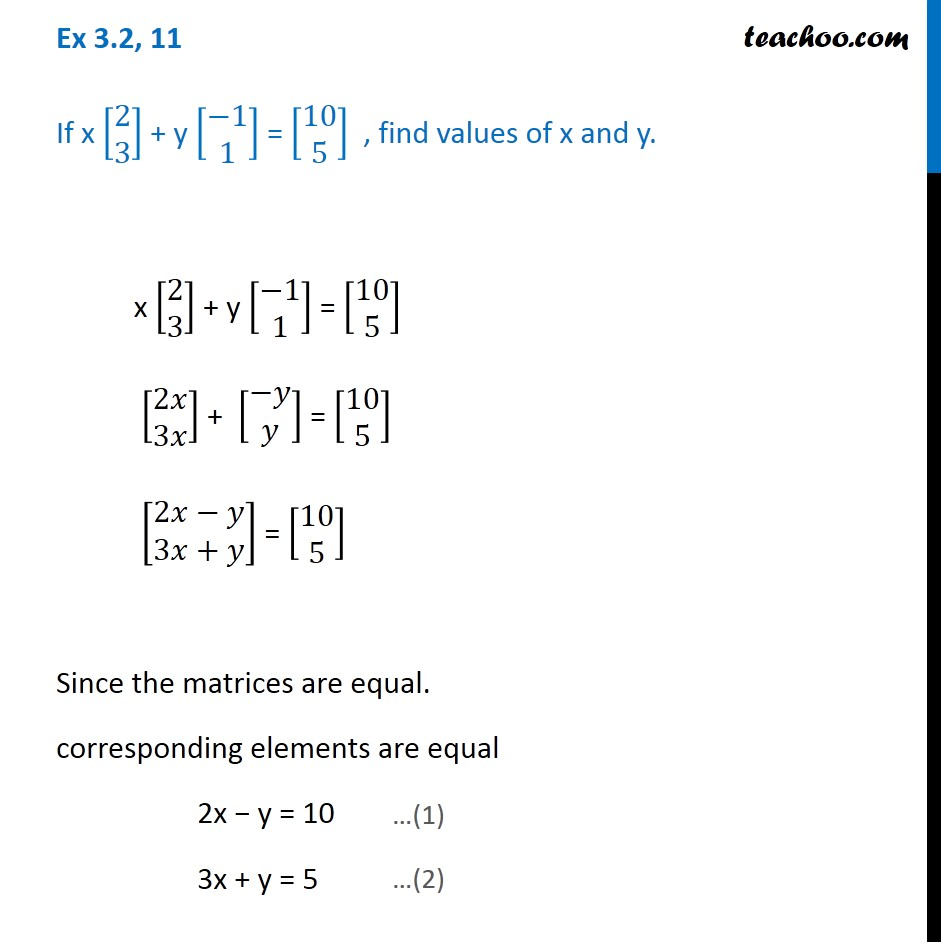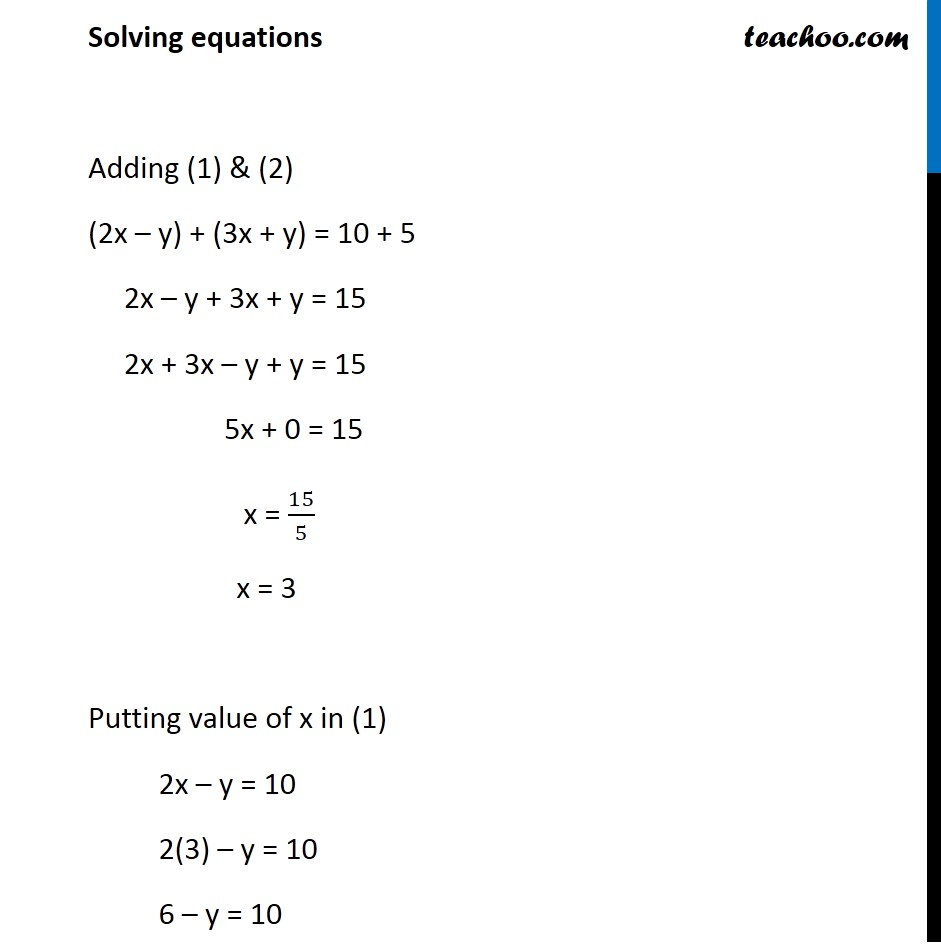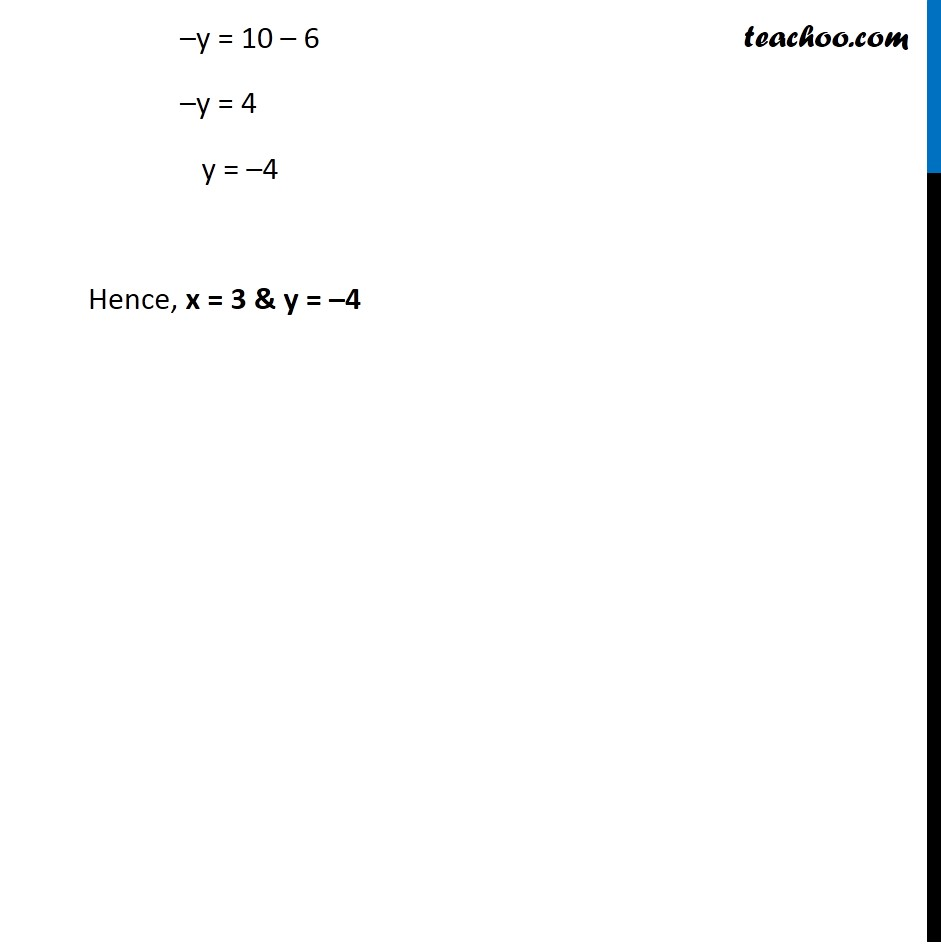Ex 3.2

Chapter 3 Class 12 Matrices
Serial order wiseGet live Maths 1-on-1 Classs - Class 6 to 12

### Transcript

Ex 3.2, 11 If x [■8([email protected])] + y [■8(−[email protected])] = [■8([email protected])] , find values of x and y. x [■8([email protected])] + y [■8(−[email protected])] = [■8([email protected])] [■8(2𝑥@3𝑥)] + [■8(−𝑦@𝑦)] = [■8([email protected])] [■8(2𝑥−𝑦@3𝑥+𝑦)] = [■8([email protected])] Since the matrices are equal. corresponding elements are equal 2x − y = 10 3x + y = 5 …(1) Solving equations Adding (1) & (2) (2x – y) + (3x + y) = 10 + 5 2x – y + 3x + y = 15 2x + 3x – y + y = 15 5x + 0 = 15 x = 15/5 x = 3 Putting value of x in (1) 2x – y = 10 2(3) – y = 10 6 – y = 10 –y = 10 – 6 –y = 4 y = –4 Hence, x = 3 & y = –4